## Main

A matrix product state (MPS) is a way of expressing a many-particle wavefunction which, for a broad class of physical states, offers a compact and accurate description with a number of parameters that increases only polynomially (that is, efficiently) in system components12. MPS tomography recognizes that the information required to identify the compact MPS is typically accessible locally; that is, by making measurements only on subsets of particles that lie in the same neighbourhood2. In such cases, the total effort required to obtain a reliable estimate for the state in the laboratory increases at most polynomially in system components2,6. States suited to MPS tomography and its generalizations to higher dimensions2 include those with a maximum distance over which significant quantum correlations exist between constituents (locally correlated states): for example, the 2D cluster states—universal resource states for quantum computing—and the ground states of a broad class of one-dimensional (1D) systems13,14,15. We find that MPS tomography is also well-suited to characterize the states generated during the dynamical evolution of systems with short-ranged interactions, as found in many physical systems.

Consider an N-component quantum system initially in a product state (or other locally correlated state) in which interactions are abruptly turned on. In the presence of finite-range interactions, information and correlations spread out in the system with a strict maximum group velocity16,17,18. Therefore, after a finite evolution time, there is a maximum distance over which correlations extend in the system (the correlation length, L), beyond which correlations decay exponentially in distance. The information required to describe the state is largely contained in the local reductions: the reduced states (density matrices) of all groups of neighbouring particles contained within L. In 1D systems, such states can be described by a compact MPS15,19 and, to identify and certify the total N-component MPS, the experimentalist need only perform the measurements required to reconstruct the local reductions (see Methods). Each local reduction can be determined by full quantum state tomography (QST), requiring measurements in at most 3L bases. Since the number of local reductions increases only linearly in N, the total number of measurement bases scales efficiently in this parameter. The local reduction estimates are passed to a classical algorithm which finds an MPS estimate in a time polynomial in N (refs 2,7) (see Fig. 1). We find that the total number of measurements required to obtain a desired fidelity for the state reconstruction also scales efficiently in N (see Methods).Figure 1: Generation and efficient characterization of locally correlated quantum states.

Our strategy is not restricted to 1D systems nor to those with strictly finite-range interactions. In any of those cases, we benefit from the fact that MPS tomography and its natural generalizations to higher spatial dimensions2 make no prior assumptions about the form of the state in the laboratory (for example, that it is pure or well described by a compact MPS), because the state estimate can be certified: an efficient assumption-free lower bound on the fidelity with the laboratory state ρlab is provided2. For example, the correlation length L need not be known a priori. If, after measurements on k sites, the certified minimum fidelity Fck between the MPS estimate |ψck〉 and the state in the laboratory ρlab is deemed not high enough, then one can try again for larger k. Generalization of our method to higher spatial dimensions and to mixed-state estimates using matrix product operators6,7 is possible, although no general certification method is currently known for mixed states20.

For finite-range interactions, the correlation length L can increase at most linearly in time as entanglement grows and spreads out in the system, demanding an exponentially growing number of measurements to estimate each local reduction21,22. This puts practical limits on the evolution time until which the system state can be efficiently characterized: once correlations have spread out over the whole system, the effort for MPS tomography becomes the same as full QST. MPS tomography is able to verify evolution towards classically intractable regimes: as the system evolves, the size of the local reductions required to obtain an accurate pure MPS description should continue to increase (as seen in our data).

Our quantum simulator consists of a string of trapped 40Ca+ ions. In each ion j = 1…N, two electronic states encode a spin-1/2 particle. Under the influence of laser-induced forces, the spin interactions are well described by an ‘XY’ model in a dominant transverse field B, with Hamiltonian HXY = i<jJij(σi+σj + σiσj+) + Bjσjz. Here Jij is an N × N spin–spin coupling matrix, σi+ (σi) is the spin raising (lowering) operator for spin i and σjz is the Pauli Z matrix for spin j. Interactions reduce approximately with a power-law Jij 1/ |ij| α with distance |ij|. Here 1.1 < α < 1.6, for which the predominant feature of spreading wave packets of correlations is nevertheless evident23,24,25. Applying MPS tomography to study complex out-of-equilibrium states, generated by interactions that are not strictly finite range, represents a most stringent test of its scope of application.

The largest application of full QST was for a simple 8-qubit W-state, employing measurements in 6561 different bases taken over ten hours26. We begin experiments with 8-spin (qubit) quench dynamics, and accurately reconstruct complex 8-spin entangled states using measurements in 27 bases taken over ten minutes. We measure in sufficient bases to reconstruct all k-local reductions of individual spins (k = 1), neighbouring spin pairs (k = 2) and spin triplets (k = 3), during simulator evolution starting from the initial highly excited Néel state |φ(0)〉 = |, , , . . .〉 (see Methods). The local measurements directly reveal important properties: single-site ‘magnetization’ shows how spin excitations disperse and then partially refocus (Fig. 2a); in the first few milliseconds, strong entanglement develops in all neighbouring spin pairs and triplets, then later reducing, first in pairs then in triplets, consistent with correlations spreading out over more spins in the system (Fig. 2c, d).

Certified fidelity lower bounds Fck ≤ 〈ψck |ρlab |ψck〉 from MPS tomography during the 8-spin quench are shown in Fig. 3a. The results closely match an idealized model where MPS tomography is applied to exact local reductions of the ideal time-evolved states |φ(t)〉 (see Methods). Measurements on k = 1 sites at t = 0 yield a certified MPS state reconstruction |ψc1〉, with Fc1 = 0.98 ± 0.01 and |〈ψc1 |φ(0)〉|2 = 0.98, proving that the system is initially well described by a pure product Néel state. As expected, the fidelity lower bounds based on single-site measurements rapidly degrade as the simulator evolves, falling to 0 by t = 2 ms. Nevertheless, an accurate MPS (pure-state) description is still achieved by measuring on larger (k = 2) and larger (k = 3) reduced sites. The model fidelity bounds Fc3 begin to drop after t = 2 ms, consistent with the time at which the information wavefronts are expected to reach next-nearest neighbours (light-like cones, Fig. 2a), allowing for correlations beyond three sites to develop. Measurements on k = 3 sites reveal an MPS description with more than 0.8 fidelity up to t = 3 ms, before the lower bound rapidly drops to 0 at 6 ms. This is consistent with the model and the entanglement properties measured directly in the local reductions (Fig. 2b, c): at t = 3 ms, entanglement in spin triplets maximizes before reducing to almost zero at 6 ms, as correlations have then spread out to include more distant spins. Beyond t = 3 ms it becomes increasingly difficult to uniquely distinguish (and certify) the global state based on 3-site local reductions (although the estimate can still be a good description).

The data in Fig. 3a clearly reveal the generation and spreading out of entanglement during simulator evolution, up to 3–4 ms, and are consistent with this behaviour continuing beyond this time. To confirm this, it would be necessary to measure on increasingly large numbers of sites, demanding measurements that grow exponentially in k. That the amount of entanglement in the simulator is growing in time can be seen from the inset in Fig. 3a: the half-chain entropies of the certified MPSs |ψc3〉 are seen to grow as expected for a sudden quench27. For all times at which Fc3 > 0 (except t = 0), the pure MPS-reconstructed states |ψc3〉 are non-separable across all partitions into contiguous blocks.

Figure 3b, c compares spin–spin correlations (‘correlation matrices’) present in |ψc3〉 at t = 3 ms (Fc3 > 0.84 ± 0.05), with those obtained directly in the lab via additional measurements. The certified MPS captures the strong pairwise correlations in the simulator state and correctly predicts the sign and spatial profile of correlations beyond next-nearest neighbour: that is, of state properties beyond those measured to construct it (beyond k = 3).

Figure 4 presents results from a 14-spin quench: far beyond the practical limits of full QST. Full QST on 14 spins would require measuring in more than 4 million bases. We reconstruct a certified MPS estimate using only 27 local measurement bases. At t14 = 4 ms, strong entanglement, in neighbouring pairs and triplets, has developed right across the system. Measurements on three sites, at t14 yield an MPS estimate |ψc3〉 with a certified minimum fidelity of Fc3 = 0.39 ± 0.08. Since Fck are only lower bounds, it is natural to ask exactly what the state fidelity is. Using the estimated 14-spin MPS state |ψc3〉 from MPS tomography, we perform direct fidelity estimation (DFE)4,5 with the experimentally generated state using an additional set of measurements, obtaining a result of 0.74 ± 0.05 (see Methods and Supplementary Fig. 10).

Clearly MPS tomography provided an accurate estimate of the 14-spin simulator state, and the fidelity lower bound of Fc3 = 0.39 ± 0.08 is correct. However, the bound is conservative and even lies quite far from the one expected from an idealized system model (0.78). We find that the certification process is compromised by errors in initial state preparation, introducing mixture at the single-spin level (see Methods). The current increase in state initialization error per spin, as our current simulator is scaled up in size, limits the ability to accurately and efficiently characterize its state. A new optical set-up yielding improved single-qubit operations should allow for a small constant error per spin beyond 20 spins.

## Methods

### Trapped-ion simulator and laser control.

We refer to the ‘axial’ direction along the ion string principal axis as z and the two ‘radial’ directions, orthogonal to the string principal axis, as the x and y axes. The frequencies of the centre-of-mass vibrational modes are: ωz = 2π × 0.214 MHz, ωy = 2π × 2.69 MHz, ωx = 2π × 2.71 MHz. Two electronic Zeeman states |S1/2, m = +1/2〉 and |D5/2, m′ = +5/2〉 in each trapped 40Ca+ ion encode the |〉 and |〉 states of a spin-1/2 particle, respectively, and are coupled by an electric quadrupole transition at 729 nm. The quantum states are coherently manipulated using a Ti:Sa CW laser with a linewidth of about 1 Hz. Two laser beam paths are employed. First, a global beam illuminates the ion string approximately equally (intensity difference between outermost and middle ion 15%), from a direction perpendicular to the ion string z axis and at an angle approximately halfway between x and y. Consider the standard Pauli spin operators σx, σy and σz. The global beam is used to implement standard frequency-resolved sideband cooling and optical pumping on the quadrupole transition, to perform σx and σy rotations simultaneously on all spins, and to realize the spin–spin interaction Hamiltonian (see later). Second, a single-ion-focused beam comes in parallel to the global beam but from the opposite direction. The direction of this beam can be switched to have its focus pointing at different ions within 12 μs, using an acousto-optic deflector. The single-ion-focused beam is frequency-detuned by about 80 MHz from the spin transition, and thereby performs an AC-Stark rotation (σz) on the ion in focus. The combination of the global resonant and the focused detuned beam enables arbitrary single-spin rotations29.

### Simulator initialization.

Each experimental sequence begins with Doppler cooling (3 ms) and optical pumping (500 μs) to initialize all N ions in the string into the |〉 state. Next, all 2N radial motional modes, transverse to the string, are cooled to the ground state via 10 frequency-resolved sideband cooling pulses (10 ms in total), followed by a second frequency-resolved optical pumping step of 500 μs. From this pure electronic and motional quantum state |N = |, , . . . 〉, the Néel state |, , . . . 〉 is created by flipping every second spin to the |〉 state. This is done using a composite pulse sequence that is robust to inhomogeneities in the laser–ion coupling strength across the string (for example, due to weak Gaussian intensity profiles). See Supplementary Section I.B. for details.

### Implementing and modelling spin–spin interactions.

Spin–spin interactions, parametrized by Jij, are realized via the global laser beam when containing three frequencies (a trichromatic beam), two of which off-resonantly drive all 2N radial vibrational modes of the string and are symmetrically detuned by ±Δ from the spin flip transition. The magnitude of the detuning |Δ| is larger than the highest radial centre-of-mass mode ωx by 2π × 79 kHz (8 spins) or 2π × 76 kHz (14 spins). The third frequency is 1 MHz detuned from the spin flip transition and compensates for AC-Stark shifts (shifts in the transition frequencies of spins across the string). When uncompensated, these AC-Stark shifts are inhomogeneous across the string and arise from the Gaussian intensity profile of the global beam coupling to far off-resonant dipole transitions. Finally, an additional overall detuning δ of all three frequencies by B = 2π × 3 kHz (8 spins) or B = 2π × 5 kHz (14 spins) generates an effective transverse field (quantified by B in HXY).

The model spin–spin coupling matrix Jij is calculated from the aforementioned ion string vibrational mode frequencies, laser detunings, ionic mass and laser–ion coupling strength (see Supplementary Methods of ref. 23). Maximum values are Jij = 2π × 25 Hz (8 spins) and Jij = 2π × 15 Hz (14 spins). For more details on the experimental implementation of the spin–spin interaction Hamiltonian see refs 23,24.

The full Hamiltonian is given by HIsing = i<jJijσixσjx + i=1N(B + Bi)σiz. That is, an Ising-type model with an overall transverse field B, set experimentally by the detuning δ. H includes small spin-dependent perturbations Bi (at the few Hz level), resulting from, for example, electric quadrupole shifts which vary along the string and magnetic field curvature across the string (in addition to our standard constant 4 G field). In the limit B |Jij|, which holds in all our experiments, the HIsing is equivalent to an XY model in a transverse field (HXY), as given in the main text.

The ideal time-evolved states can easily be calculated by brute force matrix exponentiation for 8 spins, for example, |φ(t)〉 = exp(−iHIsingt/) |φ(0)〉. For 14 spins we use two different methods. First, as a diagnostic tool to model the k-spin local reductions in the laboratory, we employ the more time-efficient Krylov subspace projection methods (Arnoldi and Lanczos processes) which, in the case of sparse Hamiltonians, give a substantial speed up and well-controlled error bounds30. Second, to calculate the full N-spin time-evolved ideal states, and compare them with the MPS estimates, we use the library function scipy.sparse.linalg.expm_multiply (ref. 31). Both methods produce equivalent states at the timescales considered in our experiments.

Regarding the model Hamiltonian HXY, all spins down |zN is the ground state, and spins pointing up |z〉 are quasiparticle excitations which disperse and scatter in the system24. The Néel state is chosen for the initial state as it is highly excited (N/2 excitations) and leads to the emergence of locally correlated entangled states involving all N particles. Furthermore, the subsequent quench dynamics evolves in a subspace whose size, contrary to those of low-excitation subspaces (the subject of our previous work ref. 23), grows exponentially with N.

### Measurements.

After preparing the initial simulator state, spin interactions are abruptly turned on (a quench) and then off after any desired evolution time t, freezing the generated state and allowing for spin measurement. At the end of every such experimental run, the state of each spin is determined individually via the standard electron shelving technique: laser light at 397 nm (and 866 nm, repumping) is sent to the ion string for several milliseconds, coupling to the strong dipole S1/2P1/2 transition. Light at 397 nm is scattered if the electron is in the |〉 state and detected using a single-ion resolving charge-coupled device (CCD) camera. Such a measurement setting corresponds to measuring each spin in the Z-basis (that is, projecting each spin into either of the two eigenstates of the Pauli σz operator). Through repeated preparation and measurement, the probabilities for single-spin outcomes, or multi-spin outcomes (correlations between spin outcomes), can be estimated. We can measure any individual spin in any single-spin basis by first implementing laser-driven spin rotations that map the eigenstates of the desired single-spin operators onto the eigenstates of the Pauli σz operator, then carrying out electron shelving as before. In this way we could, for example, measure the first spin in the Z basis, the second in X, the third in Y, and so on.

MPS tomography requires the estimation of the local reduced density matrices of all blocks of k neighbouring spins. For a 1D chain of N spins, there are Nk + 1 such blocks. We estimate each neighbouring k-spin block by measuring in 3k bases, corresponding to all combinations of projecting each spin into the eigenstates of the three Pauli operators. Each of the 3k settings has 2k distinguishable outcomes, which in total adds to 3k × 2k = 6k outcome probabilities. This set of 6k local outcome probabilities is sufficient to estimate the local k-spin reduced density matrix. Rather than measuring the Nk + 1 blocks separately, we implement a straightforward scheme to measure them at the same time, requiring a total of 3k measurements bases for the entire string (see Supplementary Section III). We carry out each of the 3k measurement settings 1,000 times. The measurement outcomes are then passed to the MPS tomography algorithm which finds an N-spin state estimate and certificate (see later) and also used directly to obtain local k-spin reduced density matrices ρ(k) (for example, Fig. 2b) via standard maximum likelihood estimation32.

### Bipartite and tripartite negativity.

The entanglement values in local reductions presented in Figs 2c, d and 4b are derived from the experimental reconstructed local states ρ(k) (maximum likelihood reconstructions), with error bars obtained via standard Monte Carlo simulations of quantum projection noise. Two ways to represent entanglement are chosen: logarithmic negativity is an entanglement measure that can be computed for a generic bipartite mixed state ρ, from the trace norm of its partial transpose ${\rho }^{{\text{T}}_{\text{A}}}$ (ref. 33) LN(ρ) = log2 ${\rho }^{{\text{T}}_{\text{A}}}$1. This expression vanishes for unentangled states and we use it to quantify the degree of entanglement in the reduced 2-qubit density matrices ρ(2) of neighbouring spin pairs: LN2(ρ(2)) = log2 ${\rho }^{{\text{T}}_{\text{A}}}$1 = log2(2 |∑nμn| + 1), where μn are the negative eigenvalues of ${\rho }^{{\text{T}}_{\text{A}}}$. For a qualitative discussion of entanglement evolution in neighbouring spin triplets ρ(3) we define tripartite logarithmic negativity LN3 as the geometric mean of the three bipartite logarithmic negativities (similar to the definition of tripartite negativity in ref. 34):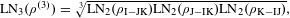where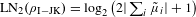, with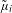as negative eigenvalues of ${\rho }^{{\text{T}}_{\text{I}}}$, the partial transpose of ρ(3) with respect to subsystem I.

### Light-like cones and interaction ranges.

Figures 2a and 4a show light-like cones representing the maximum speed at which most of the quantum information and correlations spread in our system23. Since we do not have finite-range interactions, these are not strict maximum speeds; however, they still provide a practically useful description of the spreading of correlations and information in our system23. The black lines t = d/v in Figs 2a and 4a delineate the light-like cones for a version of our system with effective nearest-neighbour interactions only (d = |iic| denotes the distance from the centre ion ic in terms of the ion sites i = 1…N). The spread velocity v is estimated as follows. We consider a nearest-neighbour-only model of our system with homogeneous coupling strength set to the average nearest-neighbour coupling of the original (full) coupling matrix Jij. We calculate the eigenmode spectrum and determine the gradient between every pair of consecutive eigenvalues. The largest of these gradients corresponds to the maximum velocity vmax at which energy and correlations disperse in the truncated system. Finally, we renormalize vmax by the algebraic tail of the original coupling matrix. Specifically, we choose the central ion ic = 5 (7) respectively for 8 (14) ions and average between the left and right algebraic tail, resulting in the normalization factor $G=\left(1/2J\right){\sum }_{{i}_{\text{c}}\ne j}\left({J}_{j,{i}_{\text{c}}}+{J}_{{i}_{\text{c}},j}\right)$ such that v = (vmax/G) with averaged nearest-neighbour interaction J (see next section). To quantify the approximate interaction range in our system we find a best fit between the eigenmode spectrum of our full Jij system model with the eigenmode spectrum of an interaction that reduces with a power-law Jij |ij| α (ref. 23). The decay parameter α yielding the best fit gives an effective interaction range of α = 1.58 (8 spins) and α = 1.27 (14 spins).

### Normalized time units (1/J).

Time axes in our plots are labelled in two ways: one way indicates the real laboratory time (in ms) passed during the evolution (for example, Fig. 2a right y-axis), while the other way shows the time normalized by the averaged nearest-neighbour interaction strength of the original coupling matrix Jij (for example, Fig. 2a left y-axis): J = (1/(N − 1))∑ i=1N−1Ji, i+1.

### Certified MPS tomography.

Here we give an overview of how we identify an MPS estimate of the unknown state in the laboratory and determine a fidelity certificate with a statistical uncertainty. In Supplementary Section IV.A, a more detailed description can be found and Supplementary Fig. 1 shows a schematic overview. The experimental data comprises the outcomes of 1000 repetitions of the 3k different measurement settings (each setting consists of a measurement basis for all N spins with distinguishable 2N outcomes). These samples are split into two parts of 500 samples each. The first part is used to obtain an MPS estimate |ψck〉 of the unknown state ρlab in the laboratory. The second part is used to obtain a certificate—that is, a lower bound on the fidelity between the unknown state ρlab and our MPS estimation |ψck〉 of this state. We split the data to ensure statistical independence of the process of obtaining the MPS estimate |ψck〉 and the process of obtaining the value of the fidelity lower bound.

The first part of the experimental data is used to estimate the 6k local outcome probabilities on each of the Nk + 1 blocks of k neighbouring spins (see Measurements above). Linear inversion is used to obtain estimates of the local k-spin reduced density matrices. These reduced density matrices are input into the modified singular value thresholding algorithm from ref. 2 to obtain a pure MPS estimate. This pure state is used as the start vector for the iterative likelihood maximization algorithm over pure states from ref. 7. Likelihood maximization uses the local outcome probabilities as input and returns an initial MPS estimate |ψest〉 of the unknown lab state. Both algorithms search for an MPS with a small bond dimension which is compatible with the local outcome probabilities. Usually, the initial estimate |ψest〉 reproduces the local outcome probabilities well, but it may or may not be close to the unknown state ρlab in the laboratory.

We certify the initial estimate |ψest〉 using a so-called parent Hamiltonian obtained from a set of candidate Hamiltonians (which may not correspond to any physical energy in the system). We compute the local reduced density matrices ρs of |ψest〉 on spins s, s + 1, …, s + k − 1. Candidate Hamiltonians are given by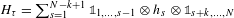, where ${P}_{ker\left({T}_{\tau }\left({\rho }_{s}\right)\right)}$ is the orthogonal projection onto the kernel of the linear operator Tτ(ρs) and Tτ replaces eigenvalues of ρs smaller than or equal to τ by zero. (If |ψest〉 is a so-called injective MPS35 then it is the non-degenerate ground state of Hτ=0 (refs 35,36). This is generally not the case for our choice of k.). For all Hamiltonians Hτ we compute a ground state |ψGS〉 and the smallest and second smallest eigenvalues E0 and E1. The typical range of E1 is the interval [0,1] (without units because Hτ is a sum of unit-less orthogonal projections). For 14 spins, we use an MPS-based ground state search12. Among all Hamiltonians Hτ with non-degenerate ground state and E1E0 > 10−6, we choose the Hamiltonian H which minimizes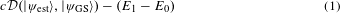where c = 5 is a constant and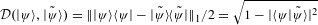is the trace distance37. We denote by H the Hamiltonian that we have chosen and by |ψck〉 the non-degenerate ground state of H; |ψck〉 is the final estimate of the unknown state in the laboratory ρlab. (If all Hτ have degenerate ground states, we do not obtain a final estimate |ψck〉 or a fidelity lower bound.) Because |ψck〉 is a ground state of H, H is called a parent Hamiltonian of |ψck〉. As the ground state |ψck〉 is non-degenerate, a lower bound to the fidelity between |ψck〉 and ρlab is given by2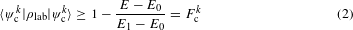where E = tr(lab) is the energy of the unknown lab state in terms of H. Knowledge of local k-spin reduced density matrices enables us to determine E because H consists of terms hs which act only on k neighbouring spins.

We use the second part of the experimental data to determine estimates of the fidelity lower bound Fck and its statistical uncertainty caused by a finite number of measurements. To estimate the statistical uncertainty, we express the energy E as weighted sum of local outcome probabilities, $E={\sum }_{s=1}^{N-k+1}{\sum }_{i=1}^{{6}^{k}}{c}_{\text{si}}{p}_{\text{si}}$. The coefficients csi are derived from the Moore–Penrose pseudoinverse of the linear map which takes a local reduced density matrix to local outcome probabilities. E is estimated using estimates of the local outcome probabilities psi. We estimate the variance of our estimate of E by estimating the covariances between each pair of local outcome probabilities. If two local outcome probabilities have been estimated from measurements in the same basis, their covariance is estimated from the measurement outcomes with a simple outcome counting scheme; otherwise, their covariance is equal to zero. Supplementary Section IV.A.4 provides full details and also considers estimating one outcome probability from more than one measurement basis.

### Ideal model of MPS tomography.

Figure 3a shows fidelity lower bounds Fck based on an ideal model of the tomographic process (dashed lines). The ideal model takes the ideal time-evolved states |φ(t)〉 = exp(−iHIsingt/) |φ(0)〉. From these states, the exact values of the 6k probabilities describing the measurement outcomes of the 3k k-fold tensor products of the Pauli X, Y and Z matrices for each of the Nk + 1 local blocks are calculated. These probabilities are used to seed the MPS tomography algorithms. Computing the energy E = tr(lab) of the (now known) ideal lab state |φ(t)〉 in terms of the parent Hamiltonian H is simplified considerably as we can compute the exact local reductions of ρlab. As a consequence, the resulting fidelity lower bounds (plotted in Fig. 3a as dashed lines) are known without uncertainty. The same model is used to obtain the idealized fidelity lower bound for the 14-spin state after 4 ms of evolution (see below). Such a numerical simulation represents a highly idealized experiment, requiring perfect initial state preparation, simulator evolution, and an infinite number of perfect measurements that would be required to obtain exact knowledge of local reductions.

In experiments, 1000 measurements per setting were performed, leading to imperfect knowledge of local reductions. The outcome of a numerical simulation of this finite number of measurements (on ideal states) is described in Supplementary Section IV.B and presented in Supplementary Fig. 2. The results show that most of the differences between the data and idealized model in Fig. 3a are due to finite measurement number effects.

### Errors in the initial simulator state.

In the main text, we state that the differences between the experimentally obtained and ideal-simulator model fidelity bounds Fc3 at t14 = 4 ms are largely explained by errors in the initial Néel state preparation for 14 spins. The initial 14-spin Néel state was prepared with a (directly measured) fidelity of 0.89 ± 0.01, compared to 0.967 ± 0.006 for the 8-spin case. This corresponds to a significantly larger error per particle for the 14-spin case (for example, error per particle can be quantified by log(F)/N, where F is the state fidelity). To determine the effect of an error in the initial state on the MPS reconstruction, we repeat the numerical simulation of the ideal-simulator model with a noisy model initial state. Out of 1000 times that we prepared and measured the initial 14-spin Néel state in the Z-basis, 893 times we observed the Néel state, 93 times we observed a state with one spin flip error and 12 times we observed two spin flip errors. The noisy model initial state is built as an appropriately weighted mixture of the ideal Néel state and single spin flip errors. At t14 = 4 ms, the fidelity lower bounds from the ideal model, the noisy model with 1000 simulated measurements and the experiment are Fc3 = 0.78, 0.49 ± 0.07 and 0.39 ± 0.08. Of course, the described errors in the initial state are not the only errors in the experiment; however, we conclude that they are largely responsible for the difference between the fidelity lower bounds obtained from experimental data and from an idealized model of a perfect simulator.

### MPS tomography is efficient for the dynamics of 1D local systems.

Certified MPS tomography can fail because no parent Hamiltonian with a non-degenerate ground state can be found. For a product state |ψ(0)〉 which evolves into |ψ(t)〉 = exp(−iHt) |ψ(0)〉 under a Hamiltonian H with one-dimensional strictly finite-range interactions, we prove the following result in Supplementary Section VII: the state |ψ(t)〉 can be certified with measurement and post-processing resources which scale, at any fixed evolution time t, polynomially in N and inverse-polynomially in 1 − FLB. N is the number of spins and FLB is the minimal permissible value of the fidelity lower bound. This means that there is a state |ψ′〉 such that the fidelity between |ψ(t)〉 and |ψ′〉 can be lower bounded by (at least) FLB using only local measurements on |ψ(t)〉. In addition, the resources required to construct |ψ′〉 obey the same polynomial scaling, as it is obtained from the ε-QCA decomposition shown in Supplementary Fig. 1138. Determining whether the certificate proof can be generalized to higher spatial dimensions will be the subject of future work.

### Direct fidelity estimation.

The fidelity lower bounds returned by the MPS certification procedure are lower bounds. That is, the actual overlap (fidelity) between the lab state ρlab (generally mixed) and the output MPS |ψc3〉 could take any value between the certificate and unity. We implement the method of direct fidelity estimation (DFE)4,5 in a 14-spin system, to estimate how big the overlap between the two states actually is.

The fidelity can be expressed as $F\left(|{\psi }_{\text{c}}^{3}〉,{\rho }_{\text{lab}}\right)=〈{\psi }_{\text{c}}^{3}|{\rho }_{\text{lab}}|{\psi }_{\text{c}}^{3}〉={\sum }_{k=1}^{{4}^{N}}{\rho }_{\text{lab}}^{k}{\sigma }^{k}$, where ρlabk = tr(Pkρlab) is the lab state’s expectation value and σk = 〈ψc3 |Pk |ψc3〉 the expectation value of the MPS |ψc3〉, with the normalized Pauli string operators Pk. The brute force approach of measuring all 4N observables is impractical for systems composed of more than a few qubits, as the number of observables to be measured increases with N. The DFE method leverages the knowledge of the MPS estimate to overcome this infeasibility: the expression above is replaced by a preferential summation over those values of k for which MPS-estimate components σk are likely to be large. In other words, more measurements are made in those Pauli operators Pk for which |ψc3〉 has a large expectation value. Therefore, we first rewrite the fidelity as the expectation value of the variable ρlabk/σk over the probability distribution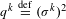: $F={\sum }_{k=1}^{{4}^{N}}{q}^{k}\left({\rho }_{\text{lab}}^{k}/{\sigma }^{k}\right)$. Next, we evaluate this using a Monte Carlo approach: we draw M random indices (k1, k2, …kM) with ki {1,2, …, 4N} according to the distribution qk and approximate the fidelity with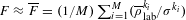. In the experiment we set M = 250 and repeat the measurements many times. Specifically, the number of copies Nk spent to measure a particular Pauli operator Pk is proportional to the inverse square of its calculated expectation value ${\sigma }^{{k}_{i}}$. In this way we prevent the error in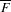from being dominated by those terms for which ${\sigma }^{{k}_{i}}$ is small. This sums to a total of 5 × 105 copies of the state. Supplementary Fig. 10a shows the experimentally measured ${\rho }_{\text{lab}}^{{k}_{i}}$ and the corresponding calculated expectation values ${\sigma }^{{k}_{i}}$ for the M = 250 different observables. Supplementary Fig. 10b depicts the distribution of ${\rho }_{\text{lab}}^{{k}_{i}}/{\sigma }^{{k}_{i}}$ for different i, from which we infer the fidelity estimate and its error: 0.74 ± 0.05. For a more detailed discussion, in particular error analysis, see Supplementary Section VI.

### Resource cost for a constant estimation error in MPS tomography.

If a quantum state of N spins in a linear chain is the only state compatible with its local reductions on k neighbouring spins, MPS tomography aims to reconstruct that state from precisely those local reductions. Reconstruction of a reduced density matrix on k spins can be achieved by measuring in the 3k bases described above. If we measure each basis on each of the Nk + 1 local blocks M times, the total number of measurements is MT = M(Nk + 1)3k. MPS tomography is efficient if it achieves a constant estimation error with a total number of measurements which scales polynomially with N. The estimation fidelity is given by F = |〈ψideal |ψest〉| 2 where |ψideal〉 is the state we want to estimate and |ψest〉 is the estimate provided by MPS tomography. We quantify the estimation error with the trace distance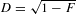between the density matrices of the two pure states.

To determine whether MPS tomography is efficient, we carried out numerical simulations on a classical computer. Specifically, we take an N-spin state |ψideal〉 that would be generated by a 3 ms quench of a nearest-neighbour model of our system with couplings and fields set to the average values of the 8-spin model. The half-chain von Neumann entropies of these states are around 0.58 (base-2 logarithm); that is, the states contain significant amounts of entanglement. On these states, we simulate M measurements in each k-spin basis as described in the last paragraph. Tomography was repeated ten times to account for fluctuations in the reconstruction error due to the finite number of measurements. Results of this simulation are shown in Supplementary Fig. 3 for N {8,20,32,48,64} spins. The left part of the figure shows that the estimation error decreases slightly with N if the ratio c = M/N2 is held constant. This means that an (at most) constant estimation error can be achieved with a total number of measurements given by MT = cN2(Nk + 1)3k, which is only cubic in N. In principle, post-processing time must also increase at least with N3, as this is how the size of the input data grows. However, with our implementation it takes only a short time to convert the input data into linearly many local expectation values. As a consequence, we observe that the time required by MPS tomography increases roughly with N1.2; in any case, post-processing time increases at most cubically with N. Hence, MPS tomography is efficient. Standard tomography is not efficient because the number of observables which have to be measured scales exponentially with N. Moreover, Supplementary Fig. 3 (right) shows that the reconstruction error of MPS tomography scales roughly linearly with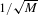, where M is the number of measurements per basis.

### Data availability.

The data that support the plots within this paper and other findings of this study are available from the corresponding author upon reasonable request.